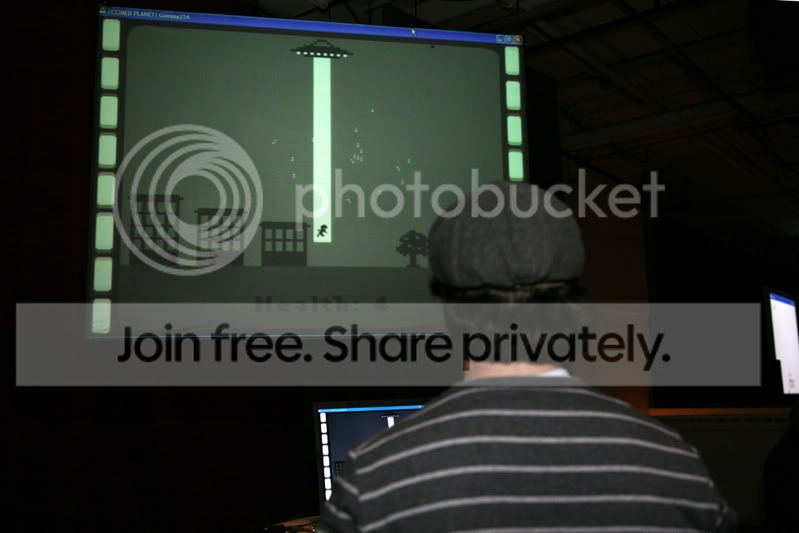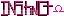-=+=- -=+=- -=+=- -=+=- -=+=- -=+=- -=+=- -=+=- -=+=- -=+=- -=+=- -=+=- -=+=- -=+=- -=+=- -=+=- -=+=- -=+=- -=+=- -=+=- -=+=- -=+=- -=+=- -=+=- -=+=- -=+=- -=+=- -=+=- -=+=- -=+=- (c) WidthPadding Industries 1987 0|309|0 -=+=- -=+=- -=+=- -=+=- -=+=- -=+=- -=+=- -=+=- -=+=- -=+=- -=+=- -=+=- -=+=- -=+=- -=+=- -=+=- -=+=- -=+=- -=+=- -=+=- -=+=- -=+=- -=+=- -=+=- -=+=- -=+=- -=+=- -=+=- -=+=- -=+=-
SoCoder -> Blogs Home -> Blogs

blanko1324Created : 02 December 2007
Edited : 02 December 2007

### Looked Like a Fun Party...Aw well, there's always next year.

Cool stuff, eh? See the rest of the pictures here.

Sunday, 02 December 2007, 15:54
JayenkaiHurraySunday, 02 December 2007, 16:23
SchererererLooks good on the big screen!Thursday, 06 December 2007, 04:56
lyons[ot]*looks at his new avatar bar*[/ot]

sorry you did not get to go , but you can always try again next year.

and i agree with instinct.